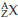×
Get Full Access to Introductory Chemistry - 5 Edition - Chapter 4 - Problem 95p
Get Full Access to Introductory Chemistry - 5 Edition - Chapter 4 - Problem 95p

×

# Write the symbol for each isotope in the form .(a) cobalt-60(b) neon-22(c) iodine-131(d)ISBN: 9780321910295 34

## Solution for problem 95P Chapter 4

Introductory Chemistry | 5th Edition

• Textbook Solutions
• 2901 Step-by-step solutions solved by professors and subject experts
• Get 24/7 help from StudySoup virtual teaching assistantsIntroductory Chemistry | 5th Edition

4 5 1 239 Reviews
28
3
Problem 95P

Write the symbol for each isotope in the form(a) cobalt-60

(b) neon-22

(c) iodine-131

(d) plutonium-244

Step-by-Step Solution:

Step 1 of 5

The symbol of an element can be written in an informative way by representing the atomic number as the subscript of the element and atomic mass of the element as the superscript of the symbol.

Step 2 of 5

Step 3 of 5

##### ISBN: 9780321910295

The answer to “?Write the symbol for each isotope in the form (a) cobalt-60(b) neon-22(c) iodine-131(d) plutonium-244” is broken down into a number of easy to follow steps, and 14 words. This textbook survival guide was created for the textbook: Introductory Chemistry, edition: 5. This full solution covers the following key subjects: cobalt, form, iodine, isotope, neon. This expansive textbook survival guide covers 19 chapters, and 2046 solutions. Since the solution to 95P from 4 chapter was answered, more than 458 students have viewed the full step-by-step answer. Introductory Chemistry was written by and is associated to the ISBN: 9780321910295. The full step-by-step solution to problem: 95P from chapter: 4 was answered by , our top Chemistry solution expert on 05/06/17, 06:45PM.

Unlock Textbook Solution